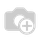# how to add days to current_date##### 2 答案

Hi willie,

You can do this by overriding the fields_view_get() of the object where you want to implement this coloring functionality:
what you can do is:

1. convert your architecture returned from calling super() from xml to dictionary :
doc = etree.fromstring(res['arch'].encode('utf8'))

2. check if 'view_type=="tree"':

3. then get new_date(date incremented from your current date)
new_due_date = datetime.now() + timedelta(days = NO_OF_DAYS)

4. create you parameter:
new_parameter = "yellow:date &gt;= '%s'"%new_due_date

5. set that parameter to color attribute of tree:
doc.set('colors', new_parameter)

6. Finally, again convert that dictionary to xml
res['arch'] = etree.tostring(doc, pretty_print=True)

7. return res

1 备注There is no way to give it directly I think. You can do something with following python code:

`import datetimecurrent_date = datetime.datetime.now().date()new_date = current_date + datetime.timedelta(days=7)`

Using that you can do it in 2 ways I think:

1. One is by overriding the fields_view_get(), which is triggered automatically on opening any views. You can refer "account_invoice.py", to understand that function and using the python code you can alter the tree view based on condition.

2. Second one to keep a boolean compute field and in the function you can check if date is greater than current date + 7. If yes, you can set it true. Call that field in tree view (hidden). For eg:

`import datetimeimport dateutil.parserfrom openerp import models, fields, apiclass sale_order(models.Model):    _inherit = 'sale.order'        check_date = fields.Boolean(compute='_check_the_date')        @api.depends('date_order')    def _check_the_date(self):        cur_date = datetime.datetime.now().date()        new_date = cur_date + datetime.timedelta(days=7)        for rec in self:            order_date = dateutil.parser.parse(rec.date_order).date()            if order_date > new_date:                rec.check_date = True`
`<record id="view_order_tree_inherited" model="ir.ui.view">            <field name="name">sale.order.tree.inherited</field>            <field name="model">sale.order</field>            <field name="inherit_id" ref="sale.view_order_tree"/>            <field name="arch" type="xml">            	<tree position="attributes">            		<attribute name="colors">green:check_date==True;grey:state=='cancel';blue:state in ('waiting_date','manual');red:state in ('invoice_except','shipping_except')</attribute>            	</tree>                <field name="state" position="after">                	<field name="check_date" invisible="1"/>                </field>            </field>        </record>`